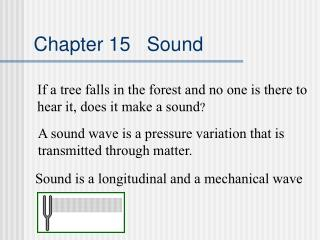DownloadDownload PresentationChapter 15 Sound

# Chapter 15 Sound

Download Presentation## Chapter 15 Sound

- - - - - - - - - - - - - - - - - - - - - - - - - - - E N D - - - - - - - - - - - - - - - - - - - - - - - - - - -
##### Presentation Transcript

1. Chapter 15 Sound If a tree falls in the forest and no one is there to hear it, does it make a sound? A sound wave is a pressure variation that is transmitted through matter. Sound is a longitudinal and a mechanical wave

2. Chapter 15 Sound The speed of sound in air at 0º C is 330 m/s and increases at .6 m/s for each degree increase in temperature. In general, the speed of sound is faster in solids and in liquids because the molecules are closer together. The frequency and the wavelength are related by the equation v = f.

3. Chapter 15 Sound Loudness: The loudness of a sound depends primarily on the amplitude of the pressure wave. Loudness is measured in decibels. Frequency: The number of cycles per second. The human ear can detect frequencies between 20 and 20,000 Hz. Frequency and loudness are not related.

4. Chapter 15 Sound Find the frequency and the period of a sound wave moving in air at room temperature (20º C) with a wavelength of .8 m. v = f or f = v/ f = (330 m/s + (.6 m/s)(20))/.8 m f = 342 m/s /.8 m= 427.5 Hz

5. Chapter 15 Sound If a sound is made and hear the echo from a wall 3 seconds later, how far away is the wall? The temperature is 30º C. d = vt d = (330 m/s + (.6 m/s)(30) ( 1.5 s) d = (348 m/s)(1.5 s) = 522 m

6. Chapter 15 Sound Doppler Shift: A change in sound frequency due to the relative motion of either the source or the detector. Demonstration

7. Chapter 15 Sound

8. Chapter 15 Sound Resonance: When the frequency of a sound matches the natural frequency of an object. Animation

9. Chapter 15 Sound

10. Chapter 15 Sound Raising and lowering the tube changes the length of the air column. When the air column is in resonance with the tuning fork, the sound is the loudest,

11. Chapter 15 Sound • Musical Instruments • String -Vibration of a string resonate a sounding board. • Brass - Vibration of lips • Reed - Vibration of a column of air • Percussion - Vibration of an object, vibrates a column of • air.

12. Chapter 15 Sound Columns of /4, 3/4, 5/4, … will all be in resonance with a tuning fork. Demonstration

13. Chapter 15 Sound Columns of /2, , 3/2, 2, … will all be in resonance with a tuning fork.

14. Chapter 15 Sound A tuning fork with a frequency of 392 Hz is found to cause resonances in an air column Spaced by 44.3 cm. The air temperature is 27º C. Find the velocity of sound in air at that temperature. Resonances are spaced by one-half wavelength so  = 2l f = 392 Hz l = 44.3 cm V = f = f(2l) = (392 Hz)(.866m) = 347 m/s

15. Chapter 15 Sound The frequency of a tuning fork is unknown. A student uses an air column at 27°C and finds resonances spaced by 39.2 cm. What is the frequency of the tuning fork? Resonances are spaced by one-half wavelength so  = 2l v = 347 m/s l = 39.2 cm F=v/  = 347m/s/.784 m = 443 Hz

16. Chapter 15 Sound Higher frequencies which are Odd-number multiples of the fundamental frequency are called Harmonics.

17. Chapter 15 Sound The ear consists of three basic parts -the outer ear, the middle ear, and the inner ear.Each part of the ear serves a specific purpose in the task of detecting and interpreting sound. The outer ear serves to collect and channel sound to the middle ear. The middle ear serves to transform the energy of a sound wave into the internal vibrations of the bone structure of the middle ear and ultimately transform these vibrations into a compressional wave in the inner ear. The inner ear serves to transform the energy of a compressional wave within the inner ear fluid into nerve impulses which can be transmitted to the brain.

18. Chapter 15 Sound

19. Chapter 15 Sound Sound Quality • Chord Several pitches played together. • Dissonance An unpleasant set of pitches. • Consonance A pleasant set of pitches. • Octave The interval between two • frequencies with a ratio of 2:1 Pythagoras noted that pleasing sounds occurred when the strings have lengths in small whole-number ratios, 1:2, 2:3, etc.

20. Chapter 15 Sound

21. Chapter 15 Sound

22. Chapter 15 Sound Beat Notes When two frequencies are close together interfere to produce high and low sounds. Fbeat= |fa - fb| Beats